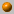+- Computational Geometry, Fall 2003/4

# Computational Geometry Fall 2003/4

Instructor: Dan Halperin , danha AT tau DOT ac DOT il
Teaching Assistant: Erez Greenstein, erezitz AT post DOT tau DOT ac DOT il

Monday, 16:00--19:00
Schreiber 006

Office hours: Wednesday, 19:00--20:00
Schreiber 219

The course covers fundamental algorithms for solving geometric problems such as computing convex hulls, intersection of line segments, Voronoi diagrams, polygon triangulation, and linear programming in low dimensional space. We will also discuss several applications of geometric algorithms to solving problems in robotics, GIS (geographic information systems), computer graphics, and more.

### Bibliography:

The main textbook of the course is
Computational Geometry: Algorithms and Applications (CGAA)
by M. de Berg, M. van Kreveld, M. Overmars, and O. Schwarzkopf.

A bibliographic list for the course.

The final grade will be determined by: 90% - a final exam, and 10% - homework assignments.

### Course Summary:

Below you'll find a very brief summary of what was covered in class during the semester. This should not be taken as a complete description of the course's contents.

For an outline of the course, see the course summary in the 1998 computational geometry course .27.10.03 Introduction

What is Computational Geometry? Course outline, motivation and techniques. Naive convex hull algorithms (see Chapter 3 in O'Rourke's book). Side-of-line test.3.11.03 Convex hull computation continued; Line segment intersection

Gift wrapping. Graham's O(n log n) algorithm (Chapter 1 in CGAA). Lower bound for convex hull algorithms.

Output sensitive algorithm to compute the intersections of line segments: Bentley Ottmann's plane sweep (Chapter 2 in CGAA).10.11.03 Sweep line; DCEL

Bentley Ottmann's plane sweep continued (Chapter 2 in CGAA). A survey of more efficient algorithms for the problem. The doubly-connected edge list (DCEL).

The last segment in CG Video Review 1992, by Tal, Chazelle, and Dobkin. Based on the paper: An optimal algorithm for intersecting line segments in the plane, by Chazelle and Edelsbrunner, J. of the ACM, Vol. 39, 1992, pp. 1--54. For recent advances on this problem see "Notes and comments" at the end of Chapter 2 of CGAA.17.11.03 Map overlay; Douglas-Peuker polyline simplefication; introduction to polygon triangulation

Overlay of planar subdivisions (CGAA, Chapter 2).

The Douglas-Peuker algorithm for line simplification. Video segment no.~1 in CG Video Review 1994, An O(n\log n) implementation of the Douglas-Peucker algorithm for line simplification, by Hershberger and Snoeyink. For details see Proc. of the 10th ACM Symposium On Computational geometry, pp. 383--384.

Introduction to triangulation (O'Rourke's book).24.11.03 Polygon triangulation; Art gallery problems

We have shown that \$\lfloor n/3 \rfloor\$ guards are sometimes necessary and always sufficient to guard a simple polygon with n vertices. We have shown that every simple polygon can be triangulated; that the triangulation graph is 3-colorable; that the dual of the triangulation graph is a tree.

A naive algorithm that mimics the triangulation existence proof can triangulate a simple polygon with n vertices in O(n^2) time. A more efficient algorithm does it in O(n\log n) by first decomposing a polygon into monotone polygons and then triangulating each of the resulting monotone subpolygons.

The material covered in class could be found in O'Rourke's book, Chapters 1 and 2, and in CGAA, Chapter 3. (The description of how to decompose a polygon into y-monotone polygons follows O'Rourke's presentation.)1.12.03 Tetrahedralization; Intersection of halfplanes; Casting;

We reviewed the results for triangulation shown in the previous lesson. Next, a few facts about tetrahedralization where surveyed: not every polyhedron is tetrahedralizable with vertices of the polyhedron only (see also Assignment no. 2); in general putting guards at the vertices of a polyhedron is insufficient for guarding the polyhedron; there are polyhedra with \$n\$ vertices that require \$\Omega(n^{3/2}))\$ guards.

A video segment from CG Video Review 1992 by Palios and Phillips: Computing a tetrahedralization of a simple polyhedron. The segment illustrates the algorithm by Chazelle and Palios, described in their paper "Triangulating a nonconvex polytope", Discrete and Computational Geometry, Vol. 5, pp. 505--526, 1990.

We have shown an \$O(n\log n)\$ algorithm for computing the intersection of \$n\$ halfplanes. This question was motivated by a problem in casting: computing whether a given polyhedron is castable. See Chapter~4 in CGAA.8.12.03 Linear Programming

We presented the linear programming problem, then a randomized incremental algorithm for LP in 2D, running in expected linear time, and finally "backward analysis" for randomized algorithms. See Chapter 4 of CGAA.15.12.03 Linear Programming cont'd; orthogonal range search

Randomized linear time algorithm for finding the minimum enclosing disc of a set of points in the plane. Sketch of LP in 3D. CGAA, Chapter 4.

Orthogonal range search I: kd-tress. CGAA, Chapter 5.22.12.03 Orthogonal range search cont'd

Planar kd-trees analysis. Planar range trees. kd-trees and range trees in fixed dimension.29.12.03 Point location

Randomized incremental construction of trapezoidal maps, and point location search structure. CGAA, Chapter 6.5.1.04 Voronoi Diagrams

Combinatorial complexity and some properties. A sweep algorithm to compute the Voronoi diagram of n points in the plane in time O(n \log n)---Fortune's algorithm. CGAA, Chapter 7.

Video segments: (i) "Visualizing Fortune's sweepline algorithm" by Seth Teller and (ii) "Moving a disc between polygons" by Stefan Schirra (both from the ACM CG Video Review 1993).

Additional references: (1) F. Aurenhammer, Voronoi diagrams: A survey of a fundamental geometric data structure , ACM Comput. Surv. 23 (1991), pp. 345--405. (2) A book full of applications---A. Okabe, B. Boots and K. Sugihara, Spatial Tessellations: Concepts and Applications of Voronoi Diagrams , John Wiley & Sons, 1992.